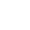﻿

/    2019-04-15

一. python 3.6 更新的新特性

1.1 f-Strings：格式化输出

1. 简单举例

``name = '太白金星'age = 18sex = '男'msg = F'姓名：{name},性别：{age}，年龄：{sex}'  # 大写字母也可以msg = f'姓名：{name},性别：{age}，年龄：{sex}'  print(msg)'''输出结果：姓名：太白金星,性别：18，年龄：男'''``

2. 任意表达式

``print(f'{3*21}')  # 63name = 'barry'print(f"全部大写：{name.upper()}")  # 全部大写：BARRY# 字典也可以teacher = {'name': '太白金星', 'age': 18}msg = f"The teacher is {teacher['name']}, aged {teacher['age']}"print(msg)  # The comedian is 太白金星, aged 18# 列表也行l1 = ['太白金星', 18]msg = f'姓名：{l1},年龄：{l1}.'print(msg)  # 姓名：太白金星,年龄：18.``

3. 可以插入表达式

``def sum_a_b(a,b):    return a + ba = 1b = 2print('求和的结果为' + f'{sum_a_b(a,b)}')``

4.多行f

``name = 'barry'age = 18ajd = 'handsome'# speaker = f'''Hi {name}.# You are {age} years old.# You are a {ajd} guy!'''speaker = f'Hi {name}.'\          f'You are {age} years old.'\          f'You are a {ajd} guy!'print(speaker)``

5.其他细节

``print(f"{{73}}")  # {73}print(f"{{{73}}}")  # {73}print(f"{{{{73}}}}")  # {{73}}m = 21# ! , : { } ;这些标点不能出现在{} 这里面。# print(f'{;12}')  # 报错# 所以使用lambda 表达式会出现一些问题。# 解决方式：可将lambda嵌套在圆括号里面解决此问题。x = 5print(f'{(lambda x: x*2) (x)}')  # 10``

f-string的格式化输出更加简洁，方便，易读。而且他的处理速度对之前的%s 或者format 有了较高的提升，所以以后尽量使用此种格式化输出。(0)

﻿